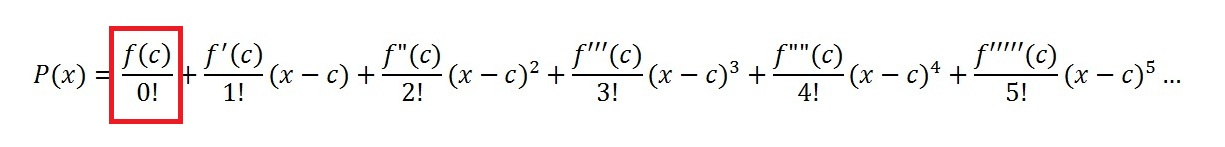# Taylor Series & Maclaurin Series with Examples

Taylor Series & Maclaurin Series help to approximate functions with a series of polynomial functions. In other words, you’re creating a function with lots of other smaller functions.

As a simple example, you can create the number 10 from smaller numbers: 1 + 2 + 3 + 4.

In the same way, you can piece together simple polynomials to create a more complicated function.

Why would you want to do this? In many cases, you know what a function looks like on a graph; but you don’t know the equation for it. The Maclaurin series can create a duplicate (a doppelgänger, if you like); it’s so close to the real thing that you won’t be able to tell the difference.

Watch the video for a definition and example of creating a Taylor series for a function:

## Taylor Series

Taylor polynomials can be used to approximate a function around any value for a differentiable function. In other words, when you use a Taylor series, you assume that you can find derivatives for your function. Taylor polynomials look a little ugly, but if you break them down into small steps, it’s actually a fast way to approximate a function.

## Example problem

Use Taylor polynomials to approximate the function cos(x) around the point x = 2.

Notes on the symbols used in the formula:

• ! is the factorial symbol).
• The “c” in the expansion is the point you’re evaluating the function at. In this example, c = 2.
• f′(x) = the first derivative.
• f′′(x) is the second derivative (i.e. take the derivative of the derivative),
• f′′′(x) is the third derivative (i.e. take the derivative of the second derivative)…and so on.
For example:

1. f(x) = x3.
2. First derivative f′(x) = 3x2;
3. Second derivative f′′(x): The derivative of 3x2 is 6x, so f′′(x) = 6x
4. Third derivative (i.e. take the derivative of the second derivative): f′′′(x) = 6.
5. …and so on.

## Steps

Step 1: Evaluate the function for the first part of the Taylor polynomial.:You’re evaluating cos(x) at x = 2, so plug in cos(2):Step 2: Evaluate the function for the second part of the Taylor polynomial.The first derivative of cos(2) is -sin(2), giving us:Step 3: Evaluate the function for the third part of the Taylor polynomial. In this step, you’re taking the second derivative (f′′(x)). In other words, we can take the derivative of the derivative we found in Step 2:Step 4: Evaluate the function for the fourth part of the Taylor polynomial. We’re finding the third derivative here, but as we found the second derivative in Step 4, we can find the derivative of that:Step 5: Continue evaluating more pieces of the Taylor polynomial, graphing the function periodically to see how well it represents your polynomial.

If we stop here, adding all of the parts from Steps 1 to 4, we get:This is what the 4th iteration looks like on a graph (graphed with Desmos):Tip: Technically, you could go on forever with iterations of the Taylor polynomial, but usually five or six iterations is sufficient for a good approximation.

## Overview

A Maclaurin series is a special case of a Taylor series, where “a” is centered around x = 0. The series are named after Scottish mathematician Colin Maclaurin. While you can calculate Maclaurin series using calculus, many series for common functions have already been found. For example, the following table shows the Maclaurin series for five common functions, along with the sigma notation for the expansion.If you don’t have one of the most common functions, the Maclaurin series is fairly easy (although somewhat tedious) to calculate.

## How to Find Maclaurin series: Steps

Example problem: Find the Maclaurin series for e5x.

Step 1: Calculate the first few derivatives for the function until you can see a clear pattern. Usually you’ll only need to calculate four or five:

 f(x)=e5x f′(x) = 5e5x f′′(x) = 52e5x f′′′(x) = 53e5x

Step 2: Fill in the derivatives you calculated in step 1 with 0 as the input. This gives you the values you need to insert into the Maclaurin series:

 f(x) = e5(0) = 1 f′(x) = 5e5(0) = 5 f′′(x) = 52e5(0) = 52 f′′′(x) = 53e5(0) = 53

Step 3: Fill in the Maclaurin formula with the values you calculated in Step 2:Step 4: (Optional): Rewrite using sigma notation:That’s how to find Maclaurin series!

CITE THIS AS:
Stephanie Glen. "Taylor Series & Maclaurin Series with Examples" From StatisticsHowTo.com: Elementary Statistics for the rest of us! https://www.statisticshowto.com/sequence-and-series/taylor-series-maclaurin-series/